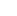# Python Code for your First Cryptocurrency Project

Create your first cryptocurrency code from scratch using the python code below. The code implements all the basic functionalities of a blockchain - from genesis block creation to securing data in blocks using a cryptographic hash function and performing transactions to ensuring blockchain immutability & transparency.

```import datetime as _dt import hashlib as _hashlib import json as _json class Blockchain: def __init__(self) -> None: self.chain = list() genesis_block = self._create_block(data = "This is genesis block", proof = 1, previous_hash = "0", index = 1 ) self.chain.append(genesis_block) def mine_block(self, data: str) -> dict: previous_block = self.get_previous_block() previous_proof = previous_block["proof"] index = len(self.chain) + 1 proof = self._proof_of_work(previous_proof, index, data) previous_hash = self._hash(block=previous_block) block = self._create_block(data=data, proof=proof, previous_hash=previous_hash, index=index) self.chain.append(block) return block def _hash(self, block: dict) -> str: encoded_block = _json.dumps(block, sort_keys=True).encode() return _hashlib.sha256(encoded_block).hexdigest() def _to_digest(self, new_proof: int, previous_proof: int, index: str, data:str) -> bytes: to_digest = str(new_proof ** 2 - previous_proof ** 2 + index) + data return to_digest.encode() def _proof_of_work(self, previous_proof: str, index: int, data: str) -> int: new_proof = 1 check_proof = False while not check_proof: to_digest = self._to_digest(new_proof=new_proof, previous_proof=previous_proof, index=index, data=data,) hash_value = _hashlib.sha256(to_digest).hexdigest() if hash_value[:4] == "0000": check_proof = True else: new_proof += 1 return new_proof def get_previous_block(self) -> dict: return self.chain[-1] def _create_block( self, data: str, proof: int, previous_hash: str, index: int) -> dict: block = { "index": index, "timestamp": str (_dt.datetime.now()), "data": data, "proof": proof, "previous_hash": previous_hash } return block def is_chain_valid(self) -> bool: current_block = self.chain block_index = 1 while block_index < len(self.chain): next_block = self.chain[block_index] if next_block["previous_hash"] != self._hash(current_block): return False current_proof = current_block["proof"] next_index, next_data, next_proof = (next_block["index"], next_block["data"], next_block["proof"]) Hash_value = _hashlib.sha256( self._to_digest(new_proof=next_proof, previous_proof=current_proof, index=next_index, data=next_data)).hexdigest() if hash_value[:4] != "0000": return False current_block = next_block block_index +=1 return True ```

To prevent data manipulation, it is crucial to consider every potential validation while developing your own cryptocurrency.

Now enter the following command into your VS's integrated terminal:

```import blockchain bc = blockchain.Blockchain() bc.mine_block("hello world") # bc.chain() ## CONFIRM THIS ```

This will output a few values that demonstrate the mining function's functionality and the working proof of work.

Run command to view the complete blockchain:

bc.chain

On your blockchain network, you will see the data for each block that has been released. The blockchain must now be mined as the following stage.

```import fastapi as _fastapi import blockchain as _blockchain blockchain = _blockchain.Blockchain() app = _fastapi.FastAPI() @app.post("/mine_block/") def mine_block(data: str): if not blockchain.is_chain_valid(): return _fastapi.HTTPException(status_code=400, detail="This blockchain is invalid") block = blockchain.mine_block(data=data) return block @app.get("/blockchain/") def get_blockchain(): if not blockchain.is_chain_valid(): return _fastapi.HTTPException(status_code=400, detail="This blockchain is invalid") chain = blockchain.chain return chain ```

Now enter localhost:8000/docs in the browser. You may even mine a block while viewing your full blockchain here. Check the validity of your blockchain code by testing it here.

To return the previous block and check whether the blockchain is legitimate, you can add a few extra endpoints to your blockchain.

```@app.get("/validate/") def is_blockchain_valid(): return blockchain.is_chain_valid() @app.get("/previous_block/") def prevoous_block(): if not blockchain.is_chain_valid(): return _fastapi.HTTPException(status_code=400, return _fastapi.HTTPException(status_code=400, detail="The blockchain is invalid") return blockchain.get_previous_block() ```

Congratulations! You’ve created your own cryptocurrency in Python.Home
Blog
Services